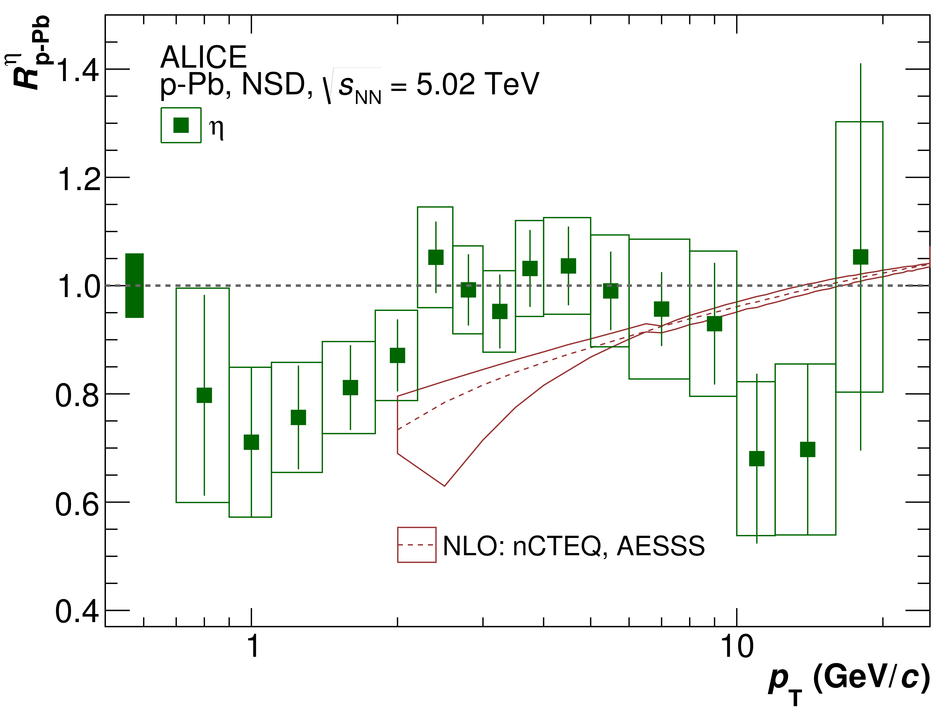Neutral pion and $η$ meson production in p-Pb collisions at $\mathbf{\sqrt{s_{\rm NN}}} = 5.02$ TeV

Neutral pion and $\eta$ meson invariant differential yields were measured in non-single diffractive p-Pb collisions at $\sqrt{s_{\rm NN}} = 5.02$ TeV with the ALICE experiment at the CERN LHC. The analysis combines results from three complementary photon measurements, utilizing the PHOS and EMCal calorimeters and the Photon Conversion Method. The invariant differential yields of $\pi^0$ and $\eta$ meson inclusive production are measured near mid-rapidity in a broad transverse momentum range of $0.3<~p_{\rm T}<~ 20$ GeV/$c$ and $0.7<~p_{\rm T}<~20$ GeV/$c$, respectively. The measured $\eta$/$\pi^{0}$ ratio increases with $p_{\rm T}$ and saturates for $p_{\rm T} > 4$ GeV/$c$ at $0.483\pm 0.015_{\rm stat}\pm 0.015_{\rm sys}$. A deviation from $m_{\rm T}$ scaling is observed for $p_{\rm T}<~2$ GeV/$c$. The measured $\eta$/$\pi^{0}$ ratio is consistent with previous measurements from proton-nucleus and pp collisions over the full $p_{\rm T}$ range. The measured $\eta$/$\pi^{0}$ ratio at high $p_{\rm T}$ also agrees within uncertainties with measurements from nucleus-nucleus collisions. The $\pi^0$ and $\eta$ yields in p-Pb relative to the scaled pp interpolated reference, $R_{\rm pPb}$, are presented for $0.3<~p_{\rm T}<~20$ GeV/$c$ and $0.7<~p_{\rm T}<~ 20$ GeV/$c$, respectively. The results are compared with theoretical model calculations. The values of $R_{\rm pPb}$ are consistent with unity for transverse momenta above 2 GeV/$c$. These results support the interpretation that the suppressed yield of neutral mesons measured in Pb-Pb collisions at LHC energies is due to parton energy loss in the hot QCD medium.

Figures

Figure 1

 The diphoton invariant mass distributions around the \pai mass for selected intervals in \pT, without and withcombinatorial background for each of the five measurements: PHOS, EMC, PCM, PCM-$\gamma^{*}\gamma$, and PCM-EMC. The vertical lines correspond to the limits of the region used to compute the integration of the meson signal.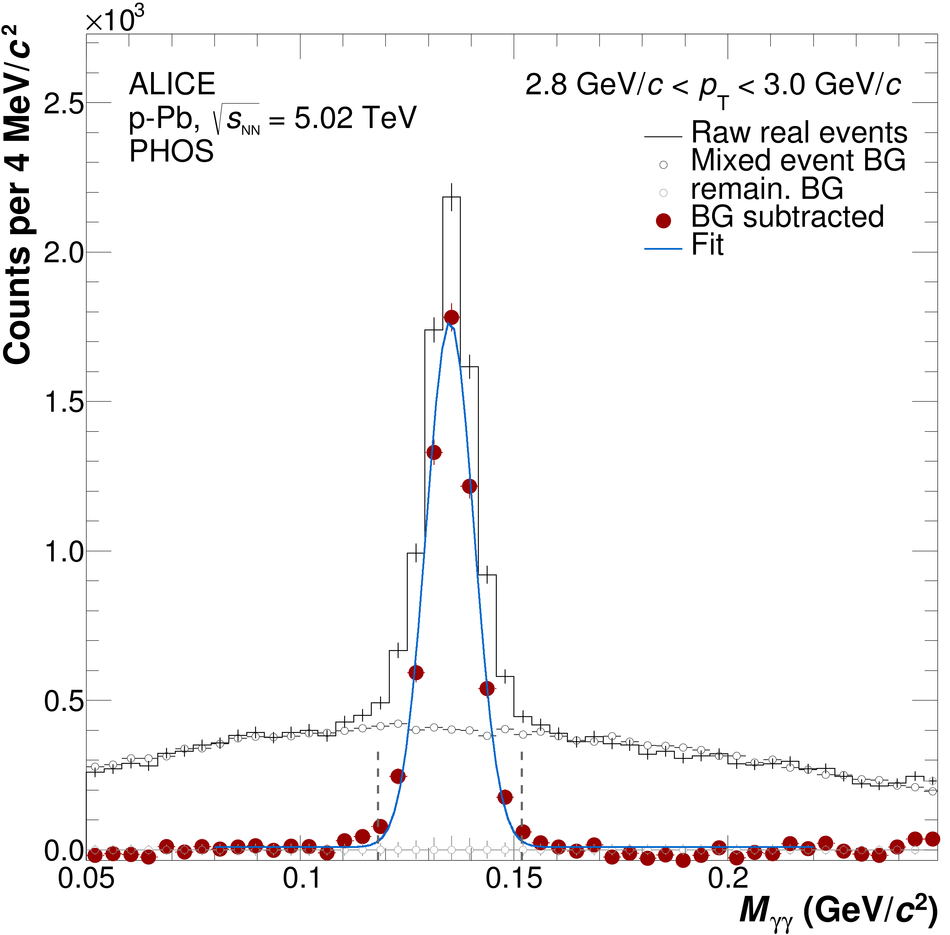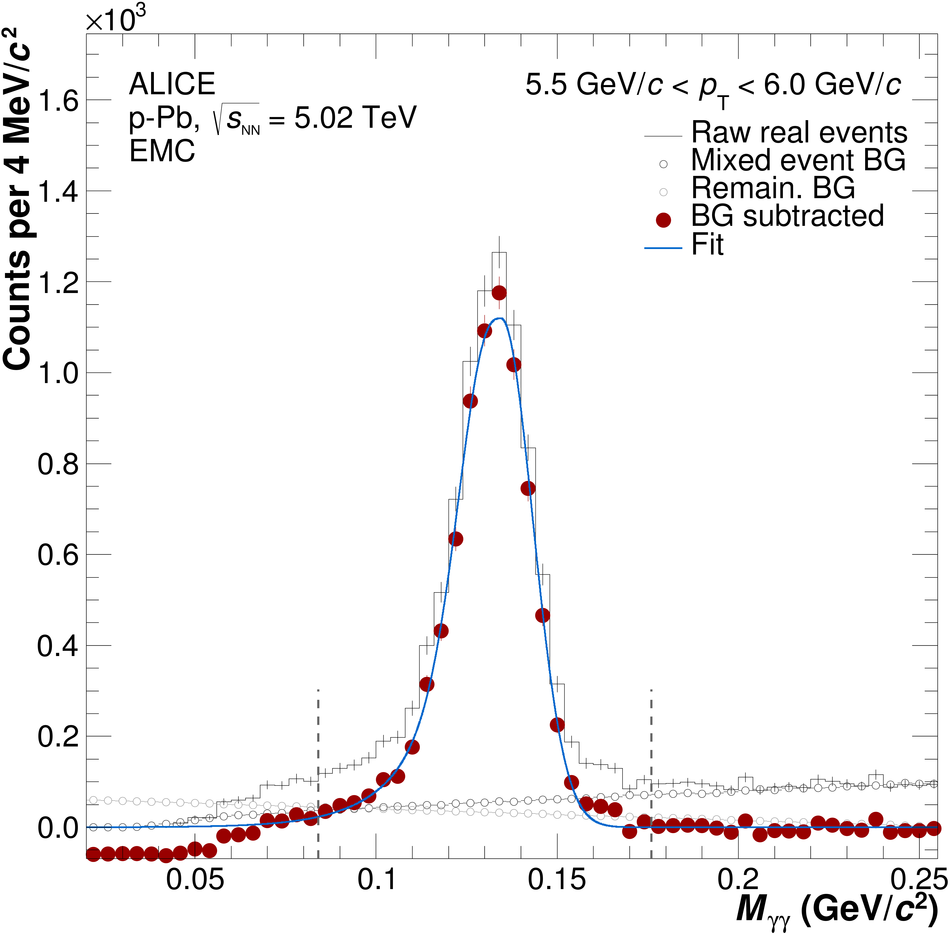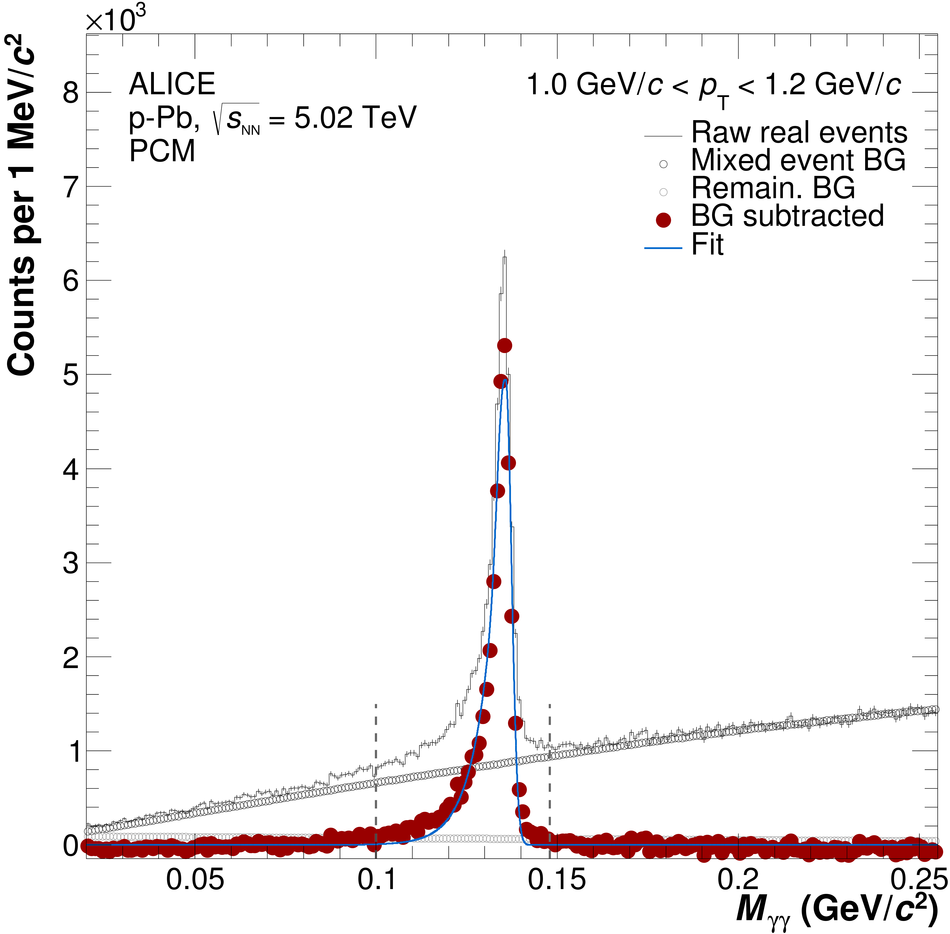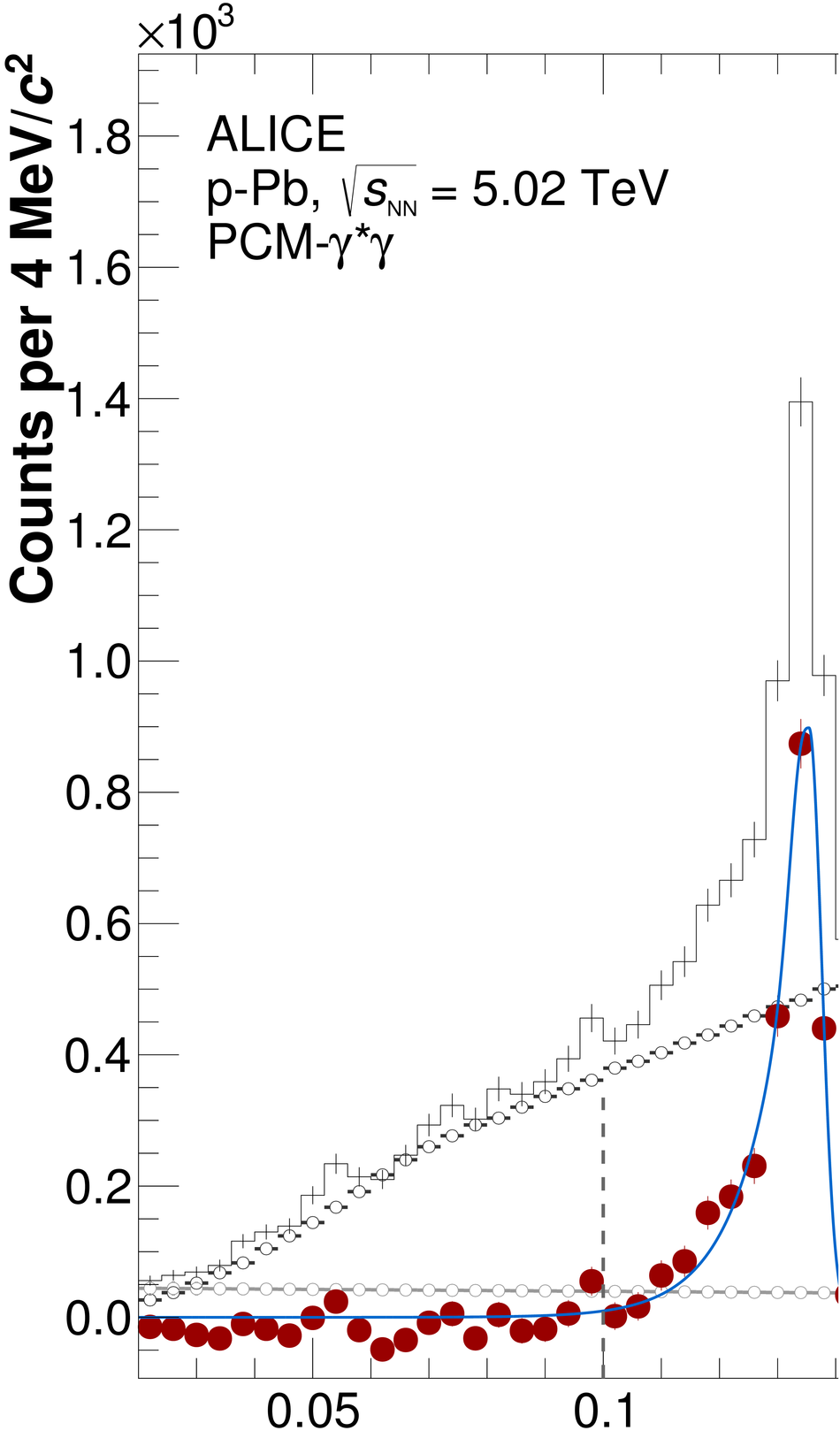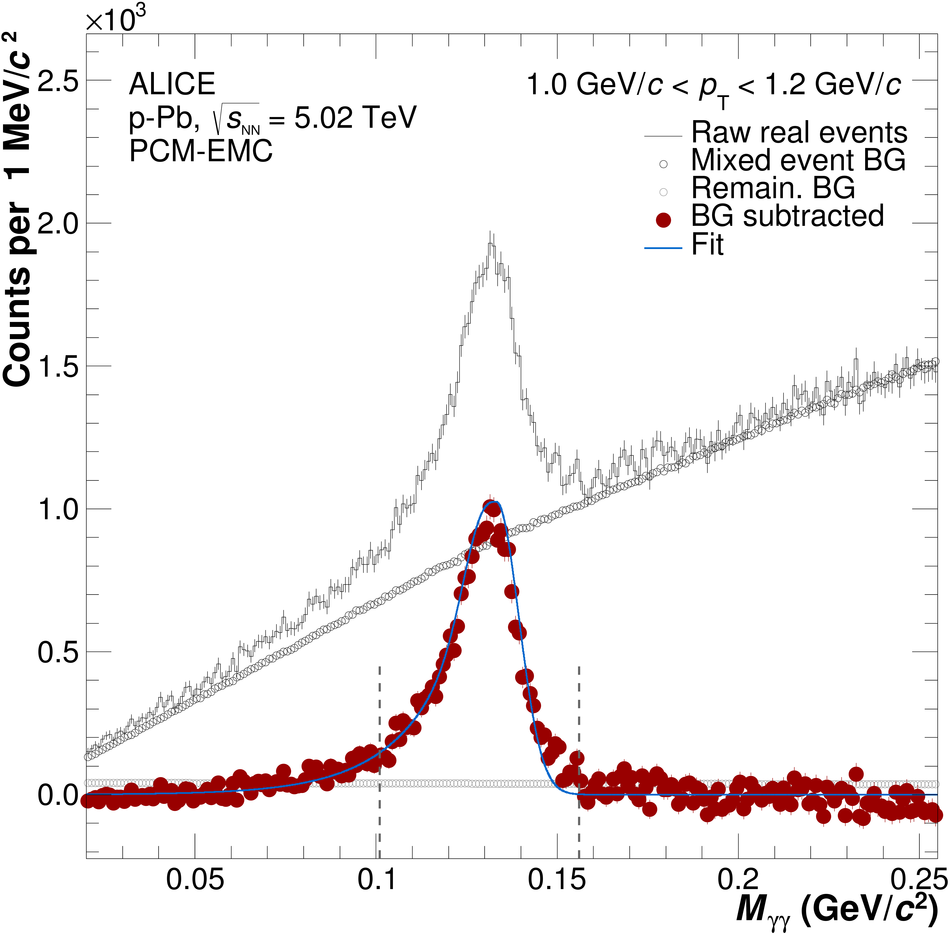Figure 2

 The diphoton invariant mass distribution around the \e mass for selected intervals in \pT, without and with combinatorial background for each of the three measurements: EMC, PCM, and PCM-EMC. The vertical lines correspond to the limits of the region used to compute the integration of the meson signal.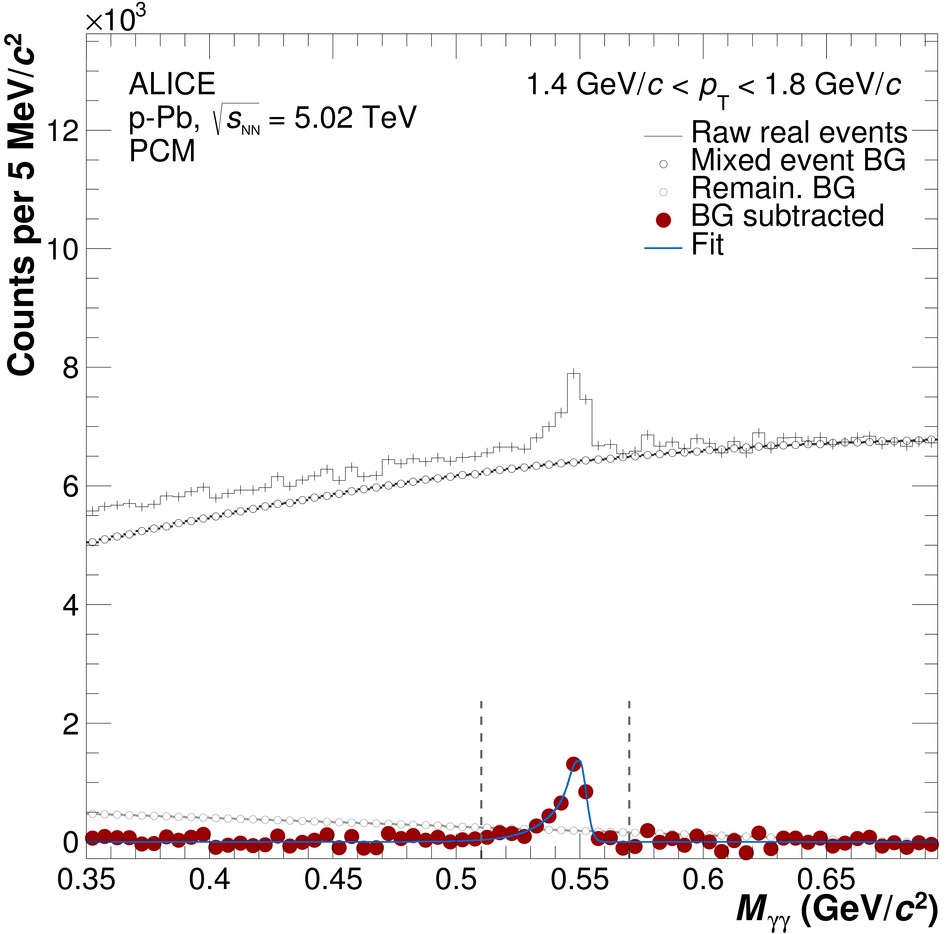Figure 3

 Reconstructed \pai mass width (top) and position (bottom) versus \pT for data and Monte Carlo simulation for all five methods.Figure 4

 Reconstructed \e mass width (top) and position (bottom) versus \pT for data and Monte Carlo simulation for PCM, EMC and PCM-EMC.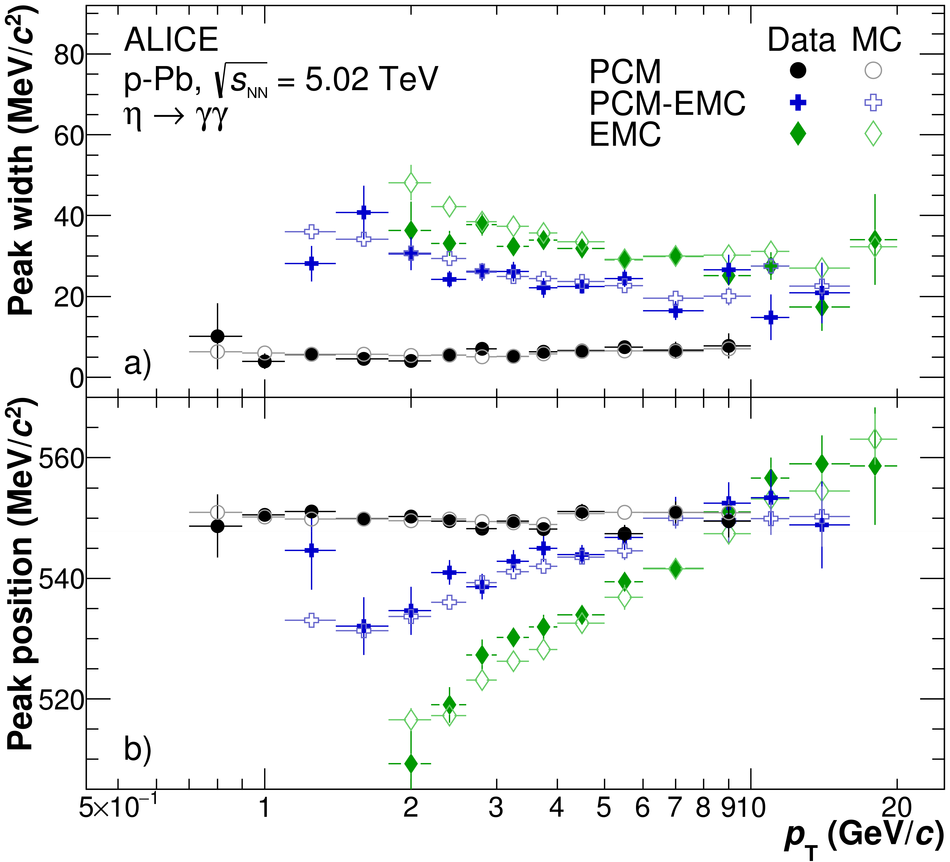Figure 5

 Left: Invariant differential \pai and \e yields produced in NSD \pPb collisions at -1.365~$< y_{\mathrm{cms}}<$~0.435 at \spPb. The statistical uncertainties are represented as vertical error bars whereas the systematic uncertainties are shown as boxes. In addition, Tsallis fits to the measured yields are shown. The TCM fit to the $\langle \TpPb \rangle$ scaled \pai and \e pp reference spectra (see \hyperref[sec:RpA]{Sect.~\ref*{sec:RpA}} for details) is shown. Right: Ratios of the measured data to their corresponding Tsallis fits.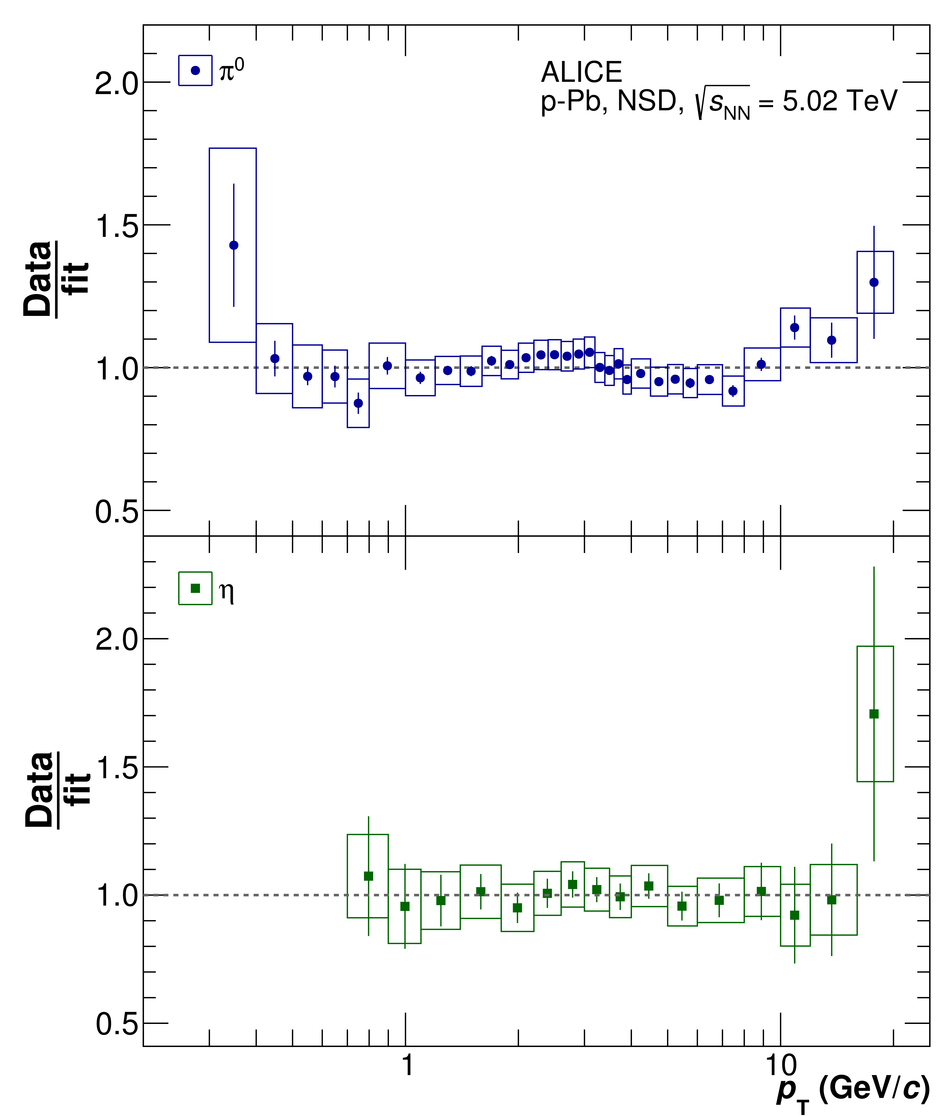Figure 6

 Ratio between individual \pai (left) and \e (right) invariant differential yield measurements, and Tsallis fit to the combined meson yield. The statistical uncertainties are represented as vertical error bars whereas the systematic uncertainties are shown as boxes.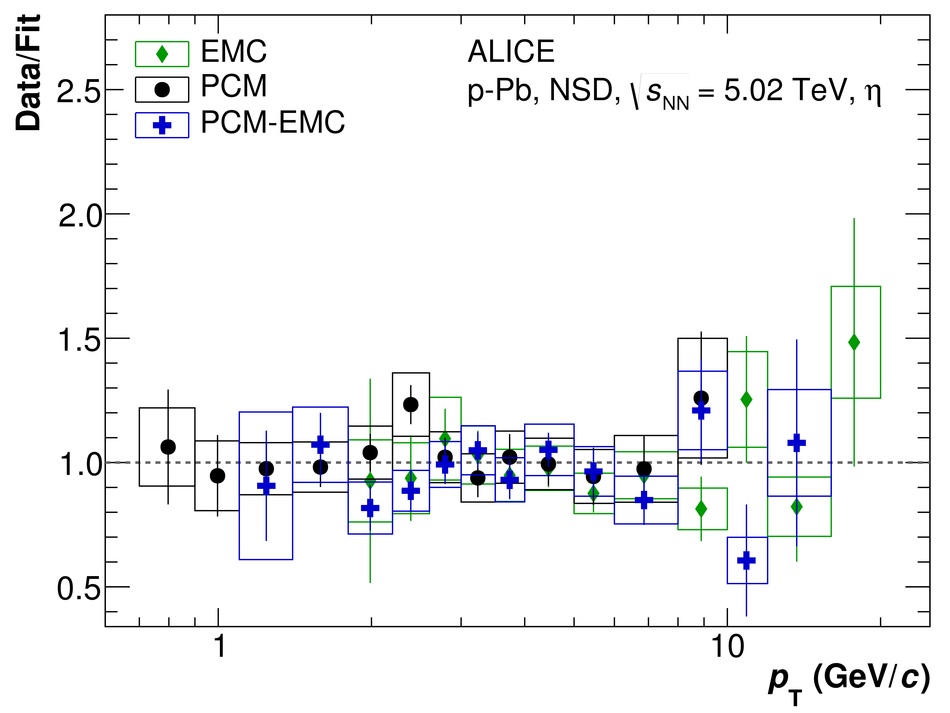Figure 7

 Left: \e /\pai ratio as function of \pT measured in NSD \pPb collisions at -1.365~$< y_{\mathrm{cms}}<$~0.435 at \spPb The statistical uncertainties are shown as vertical error bars. The systematic uncertainties are represented as boxes. For comparison, also the \e /\pai ratios measured in \unit{TeV} pp collisions with ALICE , in d-Au collisions at \sNNR with PHENIX , and in \pAu and \pBe collisions at \sNNSPS with TAPS/CERES are shown, as well as the ratio where the \e yield is obtained via \mT scaling from the measured \pPb \pai yield. Right: Ratio of the measured \e /\pai ratio to the one obtained via \mT scaling.Figure 8

 \pai (left) and \e (right) nuclear modification factors \RpPb measured in NSD \pPb collisions at -1.365~$< y_{\mathrm{cms}}<$~0.435 at \spPb compared to the nuclear modification factors of charged pions and charged kaons, respectively. The statistical uncertainties are shown as vertical error bars and the systematic uncertainties are represented as boxes. The overall normalization uncertainty is given as the solid black box around unity.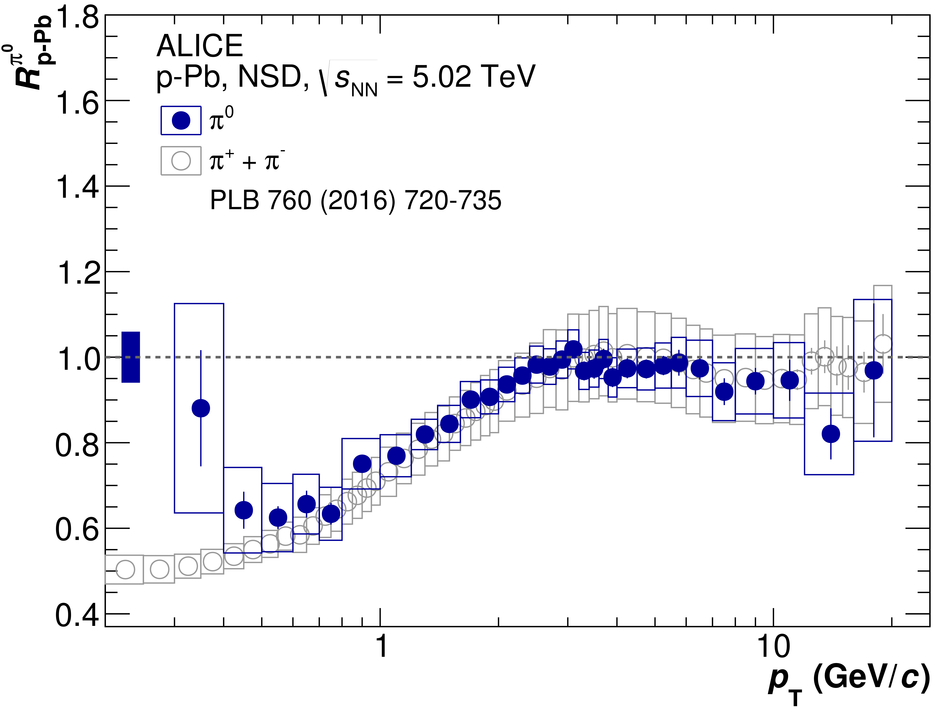Figure 9

 Comparison of several theoretical calculations to the invariant differential \pai and \e yields produced in NSD \pPb collisions at -1.365~$< y_{\mathrm{cms}}<$~0.435 at \spPb from \hyperref[fig:Pi0EtaYieldsModels]{Fig.~\ref*{fig:Pi0EtaYields}}. Theoretical calculations are shown for the EPOS3 model , CGC model , pQCD calculations at NLO using EPPS16 nPDF or using the nCTEQ nPDF and DSS14 FF for the \pai and using nCTEQ nPDF and AESSS FF for the \e meson, hydrodynamic framework (labeled as VISHNU) using the iEBE-VISHNU code , DPMJET model , and HIJING model . The blue band on the EPOS3 calculationshows the statistical errors of the prediction. The gray band on the pQCD calculation includes the uncertainties on the factorization, renormalization and fragmentation scales, as well as on the nPDF and FF The ratios of the measured data and several theoretical calculations to the data Tsallis fits (\hyperref[fig:Pi0EtaYieldsModels]{Fig.~\ref*{fig:Pi0EtaYields}}) are shown in the right panel.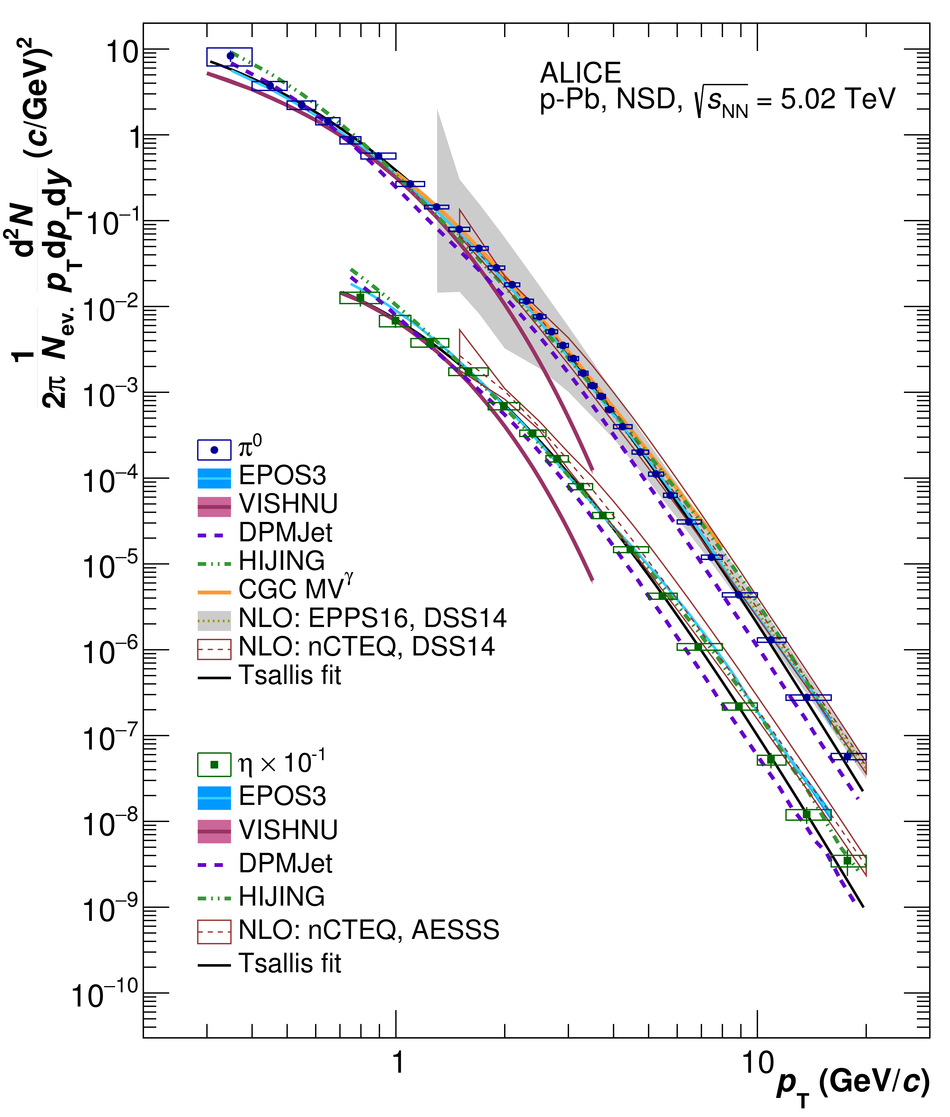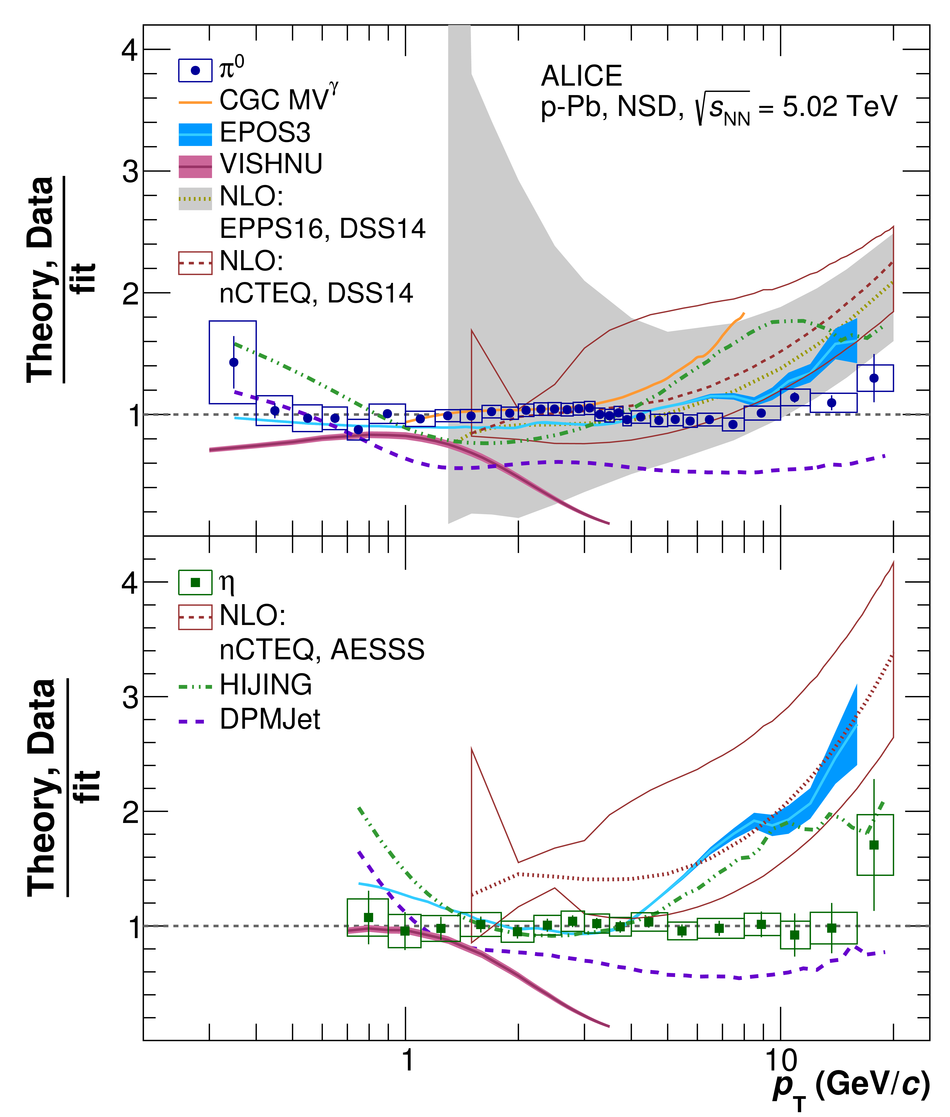Figure 10

 Comparison of different theoretical calculations to the \e /\pai ratio measured in NSD \pPb collisions at -1.365~$< y_{\mathrm{cms}}<$~0.435 at \spPb from \hyperref[fig:EtaPi0RatioALICE]{Fig.~\ref*{fig:EtaPi0RatioALICE}} Theoretical calculations are shown for the EPOS3 model with statistical errors shown as a band, hydrodynamic framework (VISHNU) using the iEBE-VISHNU code , DPMJET model and HIJING model .Figure 11

 Comparison of different theoretical model calculations to the \pai (left) and \e (right) nuclear modification factors \RpA measured in NSD \pPb collisions at -1.365~$< y_{\mathrm{cms}}<$~0.435 at \spPb. The grey band shows a pQCD calculation at NLO using the EPPS16 nPDF~, the CT14 PDF~ and the DSS14 FF~ including systematic uncertainties. Color Glass Condensate predictions using the {\ensuremath{k_{\mbox{\tiny T}}}\xspace} factorization method are also shown. NLO calculations using nCTEQ nPDF , and DSS14 FF (\pai) or AESSS FF (\e) are also shown.